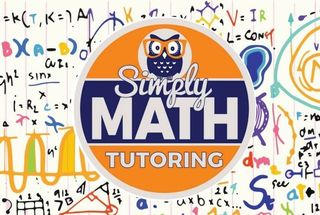\$849.00 - \$849.00

# 4 week – 7th Grade Math BootCamp• Ages:

Details of 4 week BootCamp:

Exponents

→    Understanding exponents

→    Evaluate exponents

→    Solve equations with variable exponents

→    Exponents with negative bases

→    Exponents with decimal and fractional bases

→    Evaluate numerical expressions involving exponents

Percents

→    What percentage is illustrated?

→    Convert between percents, fractions and decimals

→    Compare percents to fractions and decimals

→    Estimate percents of numbers

→    Percents of numbers and money amounts

→    Percents of numbers: word problems

Units of measurement

→    Estimate metric measurements

→    Compare and convert metric units

→    Metric mixed units

→    Convert square and cubic units of length

→    Convert between cubic metres and litres

Expressions and properties

→    Write variable expressions

→    Write variable expressions: word problems

→    Evaluate linear expressions

→    Evaluate absolute value expressions

→    Evaluate nonlinear expressions

→    Identify terms and coefficients

One-variable equations

→    Which x satisfies an equation?

→    Write an equation from words

→    Model and solve equations using algebra tiles

Two-dimensional figures

→    Identify and classify polygons

→    Name, measure and classify angles

→    Classify triangles

→    Identify trapeziums

→    Triangle angle-sum property

→    Exterior angle property

→    Find missing angles in triangles and quadrilaterals

→    Interior angles of polygons

→    Lines, line segments and half lines

→    Parallel, perpendicular and intersecting lines

Congruence and similarity

→    Similar and congruent figures

→    Side lengths and angle measures of congruent figures

→    Congruence statements and corresponding parts